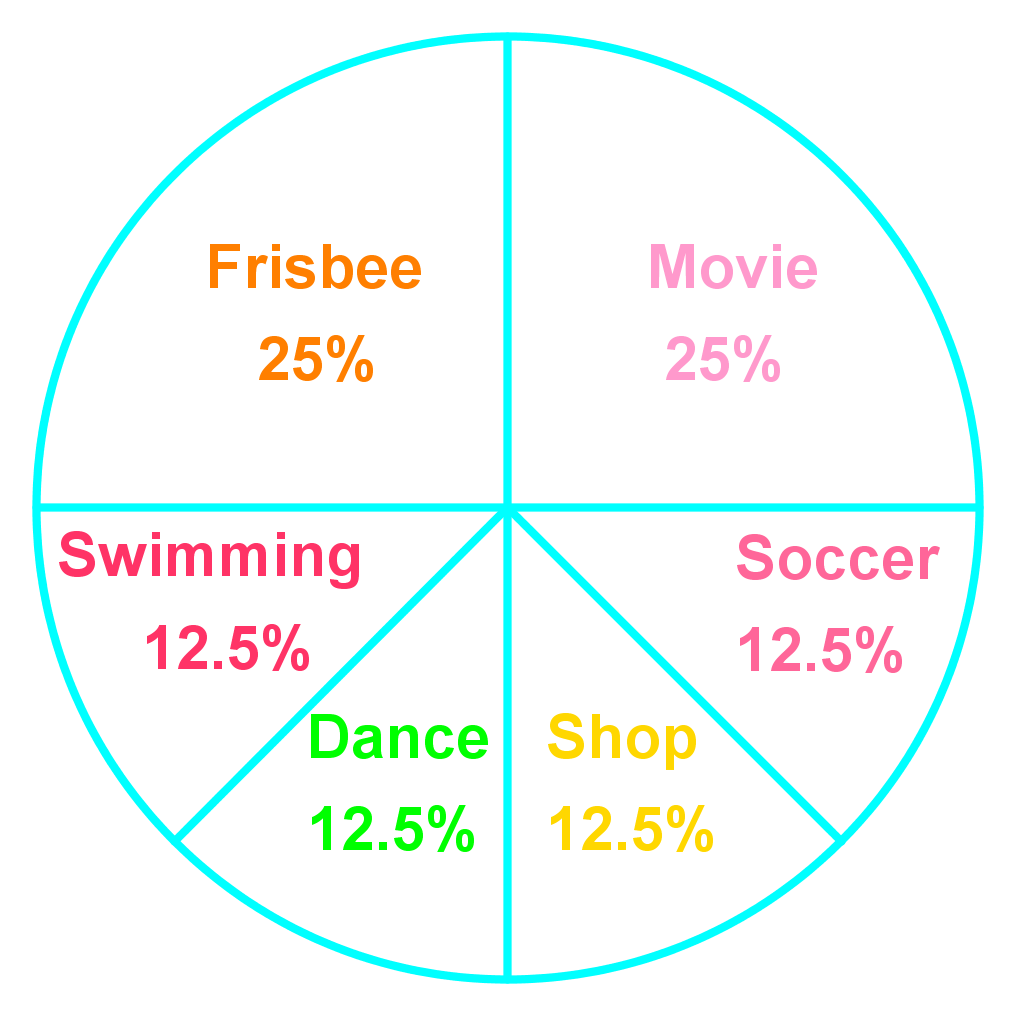# Introduction to probability#### All You Need in One Place

Everything you need for better marks in primary, GCSE, and A-level classes.#### Learn with Confidence

We’ve mastered the UK’s national curriculum so you can study with confidence.#### Instant and Unlimited Help

0/8
##### Examples
###### Lessons
1. Below is a probability model showing the weathers in fall in City A.
 Weather Probability Rain 0.35 Snow 0.08 Sunny 0.16 Cloudy 0.41

1. What is P(snow)? Write your answer as a fraction, a ratio and a percent.
2. Is it more likely to be sunny than cloudy in fall in City A?In this section, we practice finding the probability of events. Probability is the likelihood or chance of an event occurring. We will practice expressing probabilities as ratios, fractions, decimals, and percents. Probability can be calculated using the following equation: $Probability=\frac{Favourable\ outcomes}{ Possible\ outcomes}$. P(blue) is a short way to write "the probability of blue".